### Home > CALC > Chapter 5 > Lesson 5.2.4 > Problem5-95

5-95.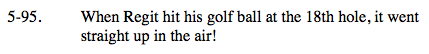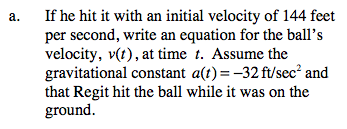$s(t)=\frac{1}{2}at^{2}+v_{0}t+s_{0}$

Find s(t). Then find v(t).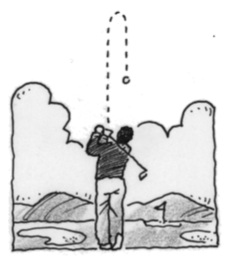s(t) = −16t² + 144t + 0
v(t) = −32t + 144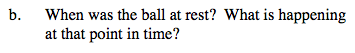The ball is at rest whenever the velocity is equal to zero. What is happening to the ball at these times?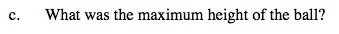Remember that the ball has a velocity of zero at the peak of its parabolic flight.